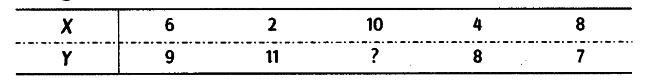# From the following data, calculate Karl Pearson's coefficient of correlation

From the following data, calculate Karl Pearson’s coefficient of correlationArithmetic mean of X and Y- series are 6 and 8, respectively.

First, we find the missing value of Y and let us denote it by a.

It indicates that there is high degree of negative correlation between X and Y.